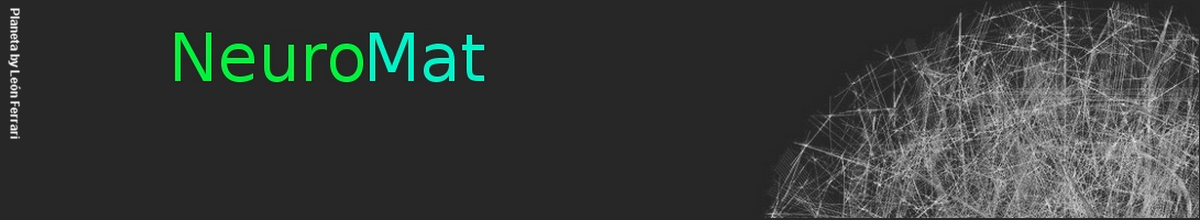### Packing arborescences in random digraphs

Carlos Hoppen, Roberto F. Parente and Cristiane M. Sato

We study the problem of packing arborescences in the random digraph D(n,p), where each possible arc is included uniformly at random with probability p=p(n). Let λ (D(n,p)) denote the largest integer λ≥0 such that, for all 0≤ℓ≤λ, we have ∑i=0ℓ−1(ℓ−i)|{v:din(v)=i}|≤ℓ. We show that the maximum number of arc-disjoint arborescences in D(n,p) is λ(D(n,p)) a.a.s. We also give tight estimates for λ(D(n,p)) depending on the range of p.

The whole paper is available here.

NeuroCineMat
Featuring this week: